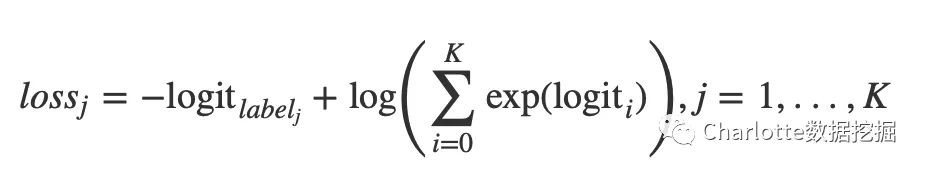# 飛槳上線萬能轉換小工具，教你玩轉TensorFlow、Caffe等模型遷移

API差異：模型的實現方式如何遷移，不同框架之間的API有沒有差異？如何避免這些差異帶來的模型效果的差異？

# TensorFlow-Fluid的API差異

``````from tensorflow.examples.tutorials.mnist import input_data
import tensorflow as tf

x = tf.placeholder(tf.float32, [None, 784])

W = tf.Variable(tf.zeros([784, 10]))
b = tf.Variable(tf.zeros())

y = tf.nn.softmax(tf.matmul(x, W) + b)

y_ = tf.placeholder("float", [None, 10])
cross_entropy = tf.reduce_sum(tf.nn.softmax_cross_entropy_with_logits(logits = y,labels = y_))

init = tf.global_variables_initializer()

sess = tf.Session()
sess.run(init)

for i in range(1, 1000):
batch_xs, batch_ys = mnist.train.next_batch(100)
sess.run(train_step, feed_dict={x: batch_xs, y_: batch_ys})

correct_prediction = tf.equal(tf.argmax(y, 1), tf.argmax(y_, 1))
accuracy = tf.reduce_mean(tf.cast(correct_prediction, 'float'))
print(sess.run(accuracy, feed_dict={x: mnist.test.images, y_: mnist.test.labels}))
``````

tf.placeholder V.S fluid.layers.data

tf.placeholder

`````` tf.placeholder(
dtype,
shape=None,
name=None
)``````

`````` paddle.fluid.layers.data(
name,
shape,
append_batch_size=True,
dtype='float32',
lod_level=0,
type=VarType.LOD_TENSOR,

Batch維度處理

TensorFlow: 對於shape中的batch維度，需要使用者使用None指定；

`````` layers.data(
name="data",
shape=[32, 3, 224, 224],
dtype="int64",
append_batch_size=False,

### 引數對比

``````tf.nn.softmax_cross_entropy_with_logits(
_sentinel=None,
labels=None,
logits=None,
dim=-1,
name=None
)
``````

``````paddle.fluid.layers.softmax_with_cross_entropy(
logits,
label,
soft_label=False,
ignore_index=-100,
numeric_stable_mode=False,
return_softmax=False
)``````

TensorFlow：labels只能使用軟標簽，其shape為[batch, num_classes]，表示樣本在各個類別上的機率分佈;

TensorFlow：傳回batch中各個樣本的log loss；

### 疑問點？numeric_stable_mode：這個引數是什麼呢？標誌位，指明是否使用一個具有更佳數學穩定性的演演算法。僅在 soft_label 為 False的GPU樣式下生效. 若 soft_label 為 True 或者執行場所為CPU, 演演算法一直具有數學穩定性。註意使用穩定演演算法時速度可能會變慢。預設為 True。

return_softmax: 指明是否額外傳回一個softmax值， 同時傳回交叉熵計算結果。預設為False。

### 程式碼示例

``````data = fluid.layers.data(name='data', shape=, dtype='float32')
label = fluid.layers.data(name='label', shape=, dtype='int64')
fc = fluid.layers.fc(input=data, size=100)
out = fluid.layers.softmax_with_cross_entropy(
logits=fc, label=label)
``````

# 模型遷移

VGG_16是CV領域的一個經典模型，我以tensorflow/models下的VGG_16為例，給大家展示如何將TensorFlow訓練好的模型轉換為飛槳模型。

``````import urllib
import sys
def schedule(a, b, c):
per = 100.0 * a * b / c
per = int(per)
sys.stderr.flush()

fetch = urllib.urlretrieve(url, "./vgg_16.tar.gz", schedule)
``````

``````import tarfile
with tarfile.open("./vgg_16.tar.gz", "r:gz") as f:
file_names = f.getnames()
for file_name in file_names:
f.extract(file_name, "./")
``````

``````import tensorflow.contrib.slim as slim
from tensorflow.contrib.slim.nets import vgg
import tensorflow as tf
import numpy

with tf.Session() as sess:
inputs = tf.placeholder(dtype=tf.float32, shape=[None, 224, 224, 3], name="inputs")
logits, endpoint = vgg.vgg_16(inputs, num_classes=1000, is_training=False)
``````
`````` numpy.random.seed(13)
data = numpy.random.rand(5, 224, 224, 3)
input_tensor = sess.graph.get_tensor_by_name("inputs:0")
output_tensor = sess.graph.get_tensor_by_name("vgg_16/fc8/squeezed:0")
result = sess.run([output_tensor], {input_tensor:data})
numpy.save("tensorflow.npy", numpy.array(result))
``````
``````    saver = tf.train.Saver()
saver.save(sess, "./checkpoint/model")``````

TensorFlow2fluid目前支援checkpoint格式的模型或者是將網路結構和引數序列化的pb格式模型，上面下載的vgg_16.ckpt僅僅儲存了模型引數，因此我們需要重新載入引數，並將網路結構和引數一起儲存為checkpoint模型

### 將模型轉換為飛槳模型

``````import tf2fluid.convert as convert
import argparse
parser = convert._get_parser()
parser.meta_file = "checkpoint/model.meta"
parser.ckpt_dir = "checkpoint"
parser.in_nodes = ["inputs"]
parser.input_shape = ["None,224,224,3"]
parser.output_nodes = ["vgg_16/fc8/squeezed"]
parser.use_cuda = "True"
parser.input_format = "NHWC"

convert.run(parser)
``````

``````INFO:root:Loading tensorflow model...
INFO:tensorflow:Restoring parameters from checkpoint/model
INFO:tensorflow:Restoring parameters from checkpoint/model
INFO:root:TotalNum:86,TraslatedNum:1,CurrentNode:inputs
INFO:root:TotalNum:86,TraslatedNum:2,CurrentNode:vgg_16/conv1/conv1_1/weights
INFO:root:TotalNum:86,TraslatedNum:3,CurrentNode:vgg_16/conv1/conv1_1/biases
INFO:root:TotalNum:86,TraslatedNum:4,CurrentNode:vgg_16/conv1/conv1_2/weights
INFO:root:TotalNum:86,TraslatedNum:5,CurrentNode:vgg_16/conv1/conv1_2/biases
...
INFO:root:TotalNum:86,TraslatedNum:10,CurrentNode:vgg_16/conv3/conv3_1/weights
INFO:root:TotalNum:86,TraslatedNum:11,CurrentNode:vgg_16/conv3/conv3_1/biases
INFO:root:TotalNum:86,TraslatedNum:12,CurrentNode:vgg_16/conv3/conv3_2/weights
INFO:root:TotalNum:86,TraslatedNum:13,CurrentNode:vgg_16/conv3/conv3_2/biases

INFO:root:TotalNum:86,TraslatedNum:86,CurrentNode:vgg_16/fc8/squeezed
INFO:root:Model translated!
``````

### 預測結果差異

``````import numpy

numpy.random.seed(13)
data = numpy.random.rand(5, 224, 224, 3).astype("float32")
# NHWC -> NCHW
data = numpy.transpose(data, (0, 3, 1, 2))

results = model.inference(feed_dict={model.inputs:data})

``````

### 對比模型損失

``````import numpy
print(numpy.max(diff))
``````

### 列印輸出

``````6.67572e-06
``````

### 需要註意的點

1. 轉換後的模型需要註意輸入格式，飛槳中輸入格式需為NCHW格式。此例中不涉及到輸入中間層，如摺積層的輸出，需要瞭解的是飛槳中的摺積層輸出，摺積核的shape與TensorFlow有差異。

2. 模型轉換完後，檢查轉換前後模型的diff，需要測試得到的最大diff是否滿足轉換需求。